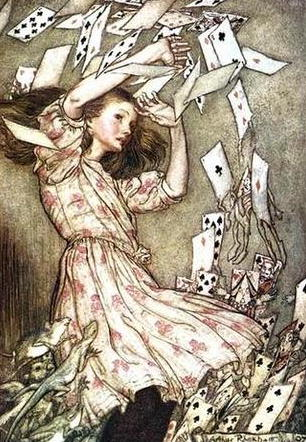Art by Arthur Rackham

The Cheshire Cat thinks of a number between $1$ and $n$ and asks Alice to guess the number as quickly as possible using arbitrary yes/no questions. From her experience in cryptography, Alice has learnt about binary search and is thus confident that she can solve the challenge with $\lceil\log n\rceil$ questions.

However, the Cheshire Cat is known to interpret ‘truthfulness’ a little unconventionally and might lie up to once during the game.

Now Alice could ask every question twice to force the cat into answering correctly, however she’d rather not risk annoying the cat by consuming $2\log n$ of its time steps.

But is there a better strategy?

## A Strategy for Alice

As it is often the case with these riddles, it turns out there is such a strategy and in fact it is very simple.

Alice begins by determining the bits of the secret number using $\log n$ questions. Next she finds out whether the cat lied already by asking some constant number of times whether this is the correct number. If the cat wants to play as long as possible, it will of course have lied and one of the bits Alice has is wrong. However, it is now very easy for Alice to figure out which bit is wrong by performing a binary search on the bits. Note that it is not very difficult to generalise this for the case where $k$ lies are allowed.

This strategy needs

$\log n + \log \log n + O(1)$

questions. A proof by hand-waving shows this to be a lower bound too.

## Further Remarks

This game is known as Rényi-Ulam’s game and got some attention in coding theory. Apparently it is rather difficult to find the exact number of questions needed or rigorously show lower bounds. There is a wikipedia article with further references.

CC-BY-SA Adrian Neumann (PGP Key A0A8BC98)